# RD Sharma Solutions for Class 10 Maths Chapter 4 Triangles Exercise 4.5

There are several criteria for proving the similarity of triangles. All these criteria are discussed in this exercise. Students who want to understand these criteria in detail can refer to the RD Sharma Solutions Class 10 for securing high or full marks in the examinations. Also, make use of the RD Sharma Solutions for Class 10 Maths Chapter 4 Triangles Exercise 4.5 PDF provided below for further assistance.

## RD Sharma Solutions for Class 10 Maths Chapter 4 Triangles Exercise 4.5 Download PDF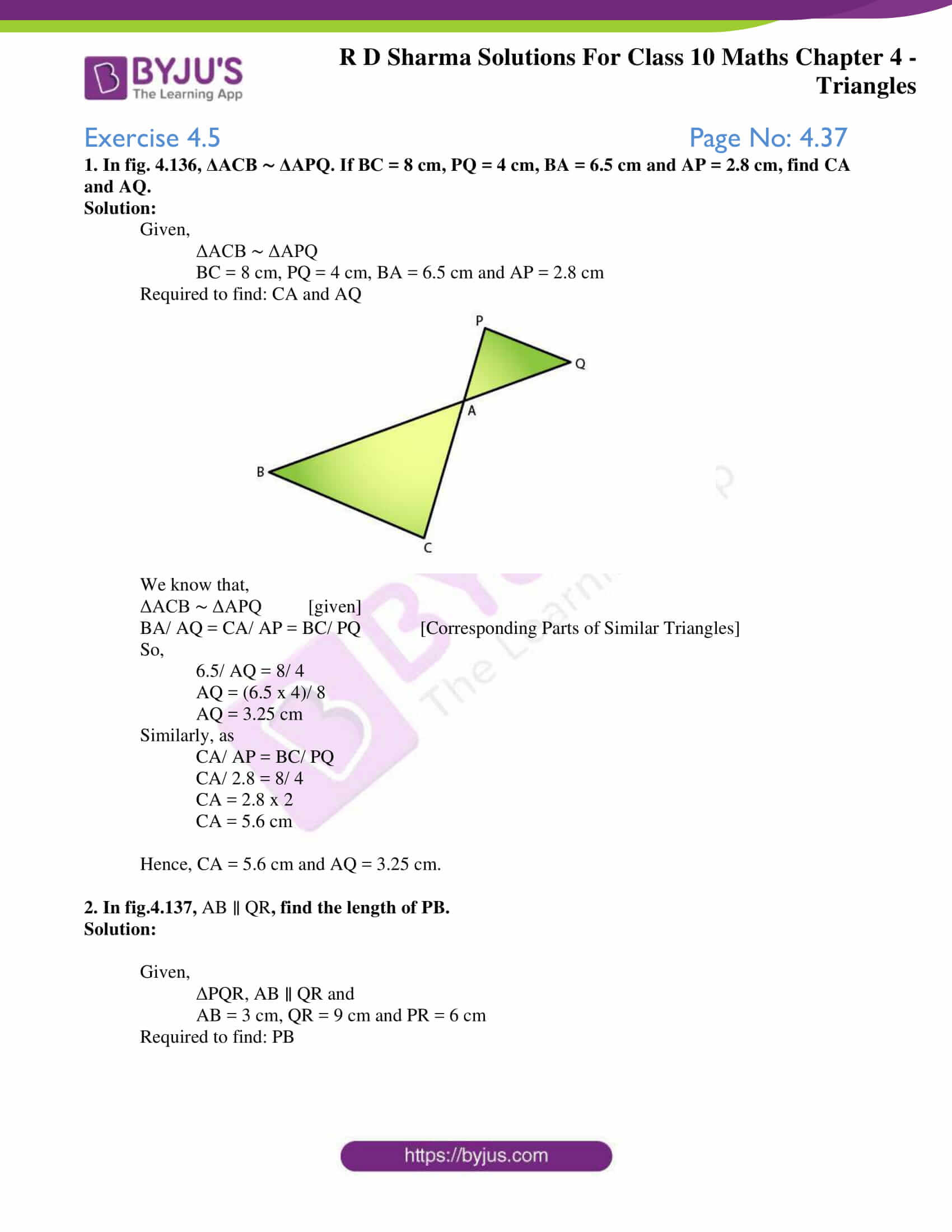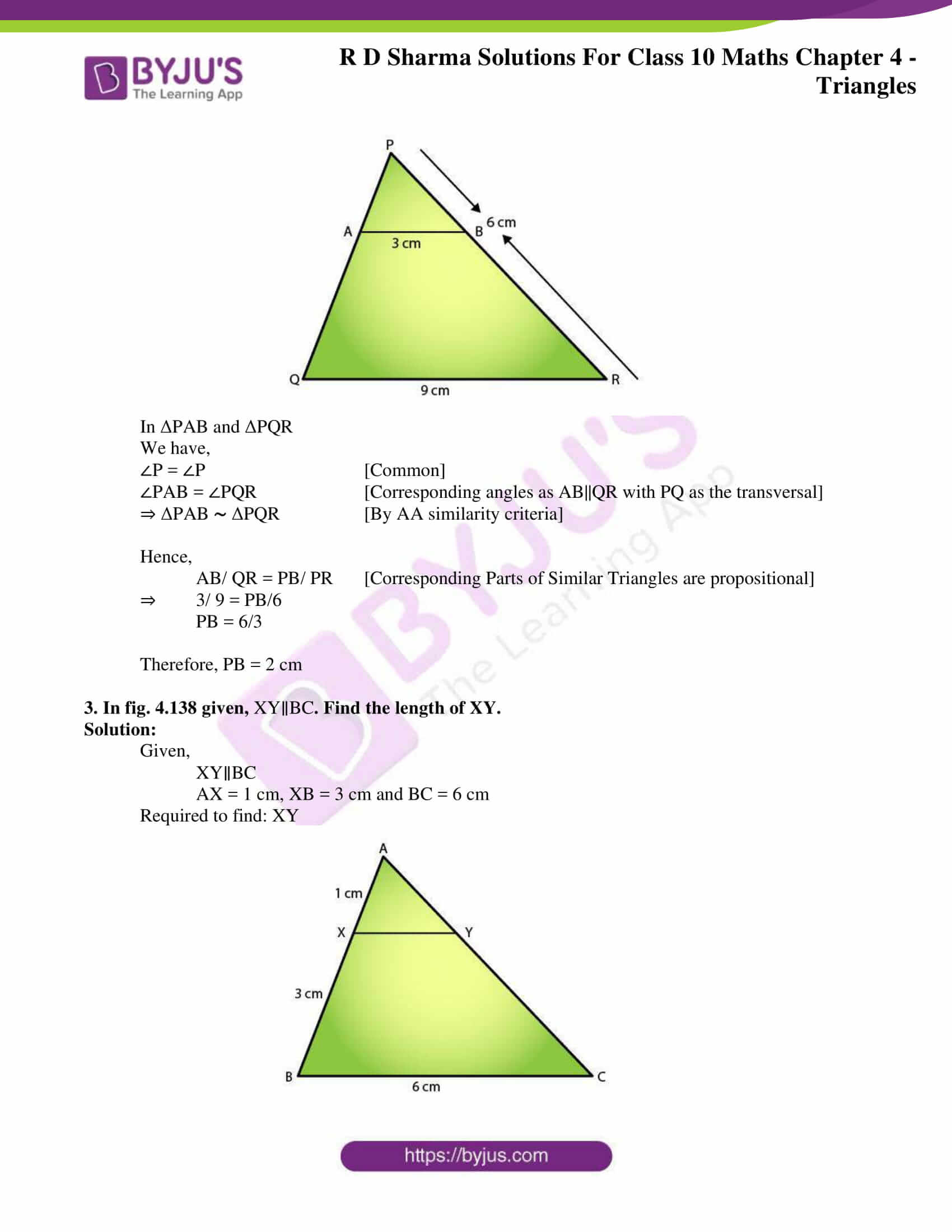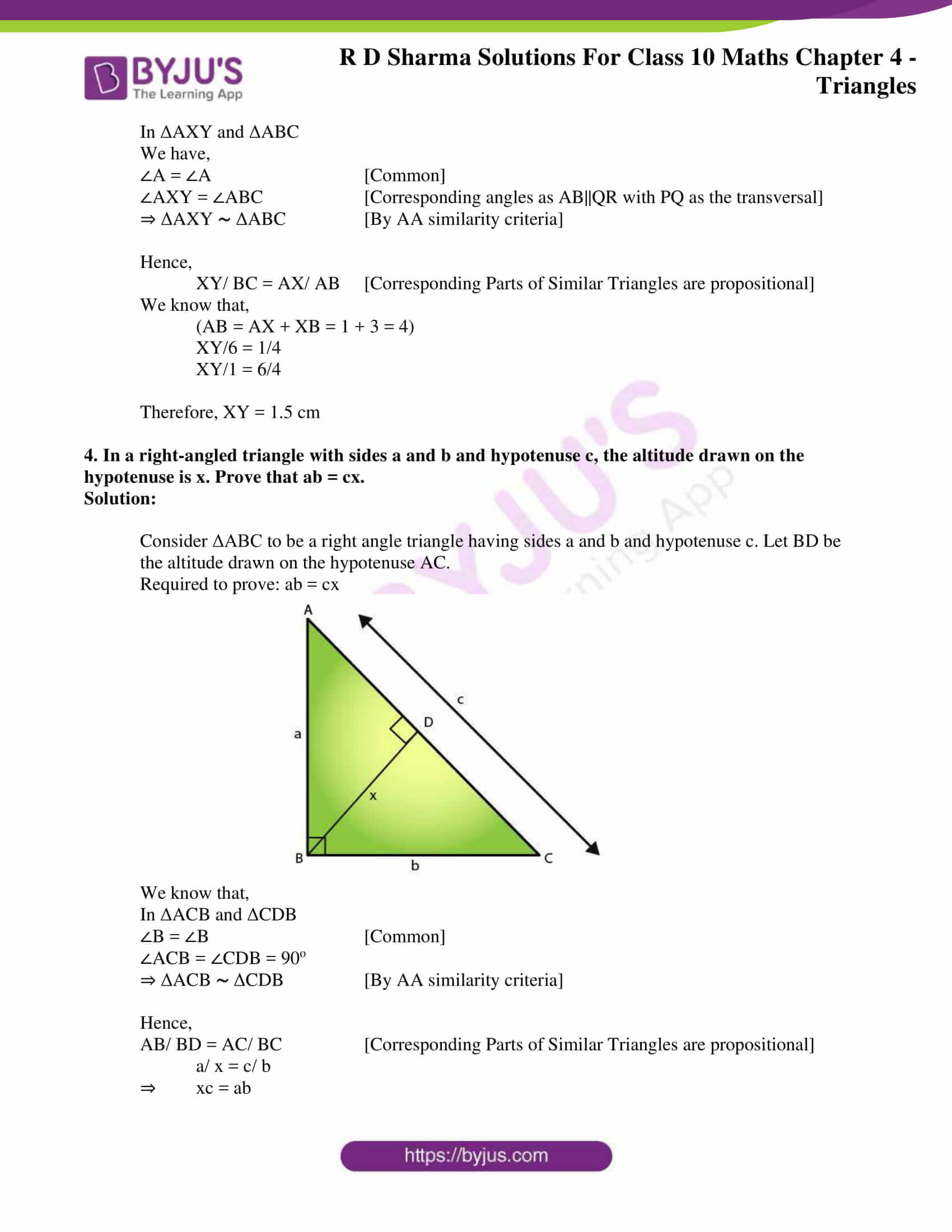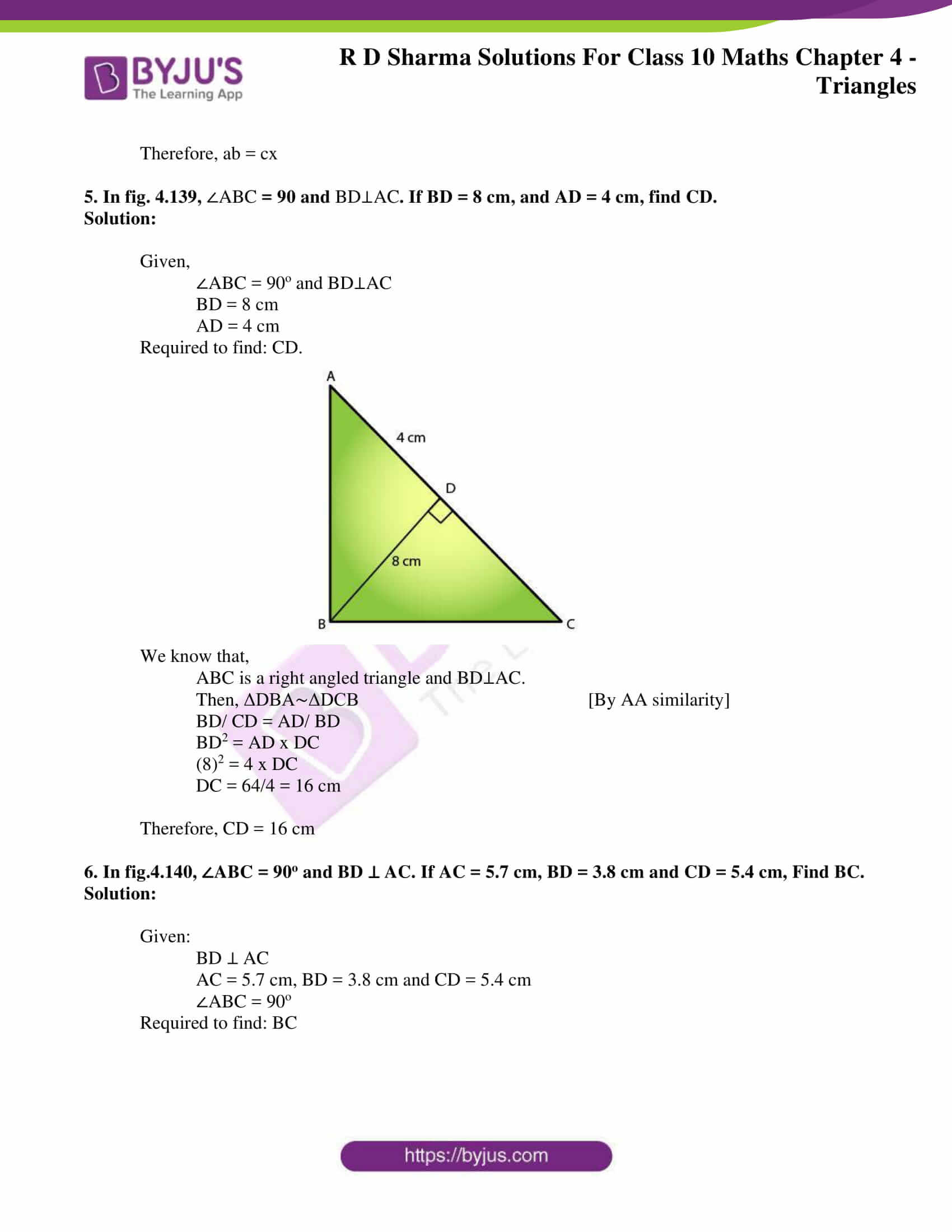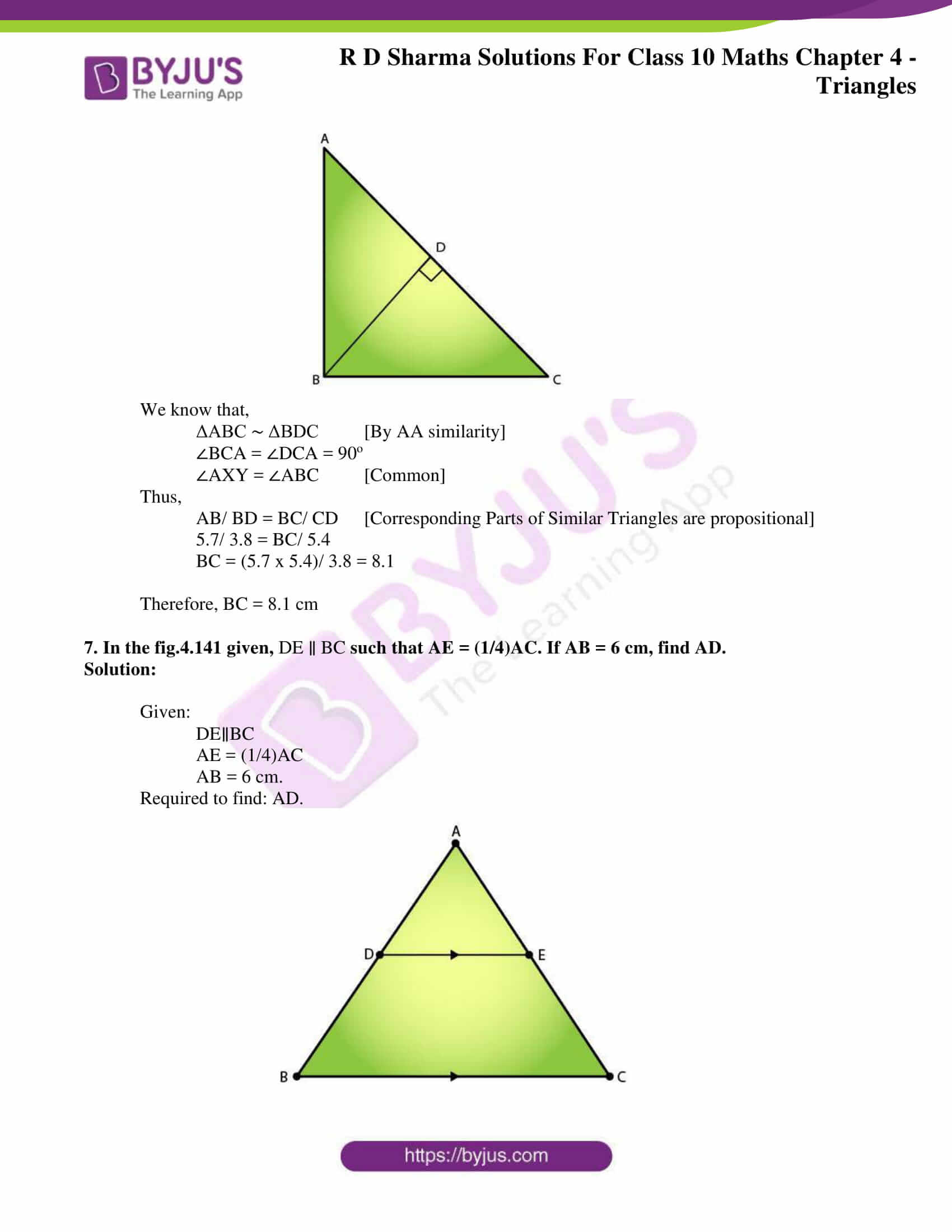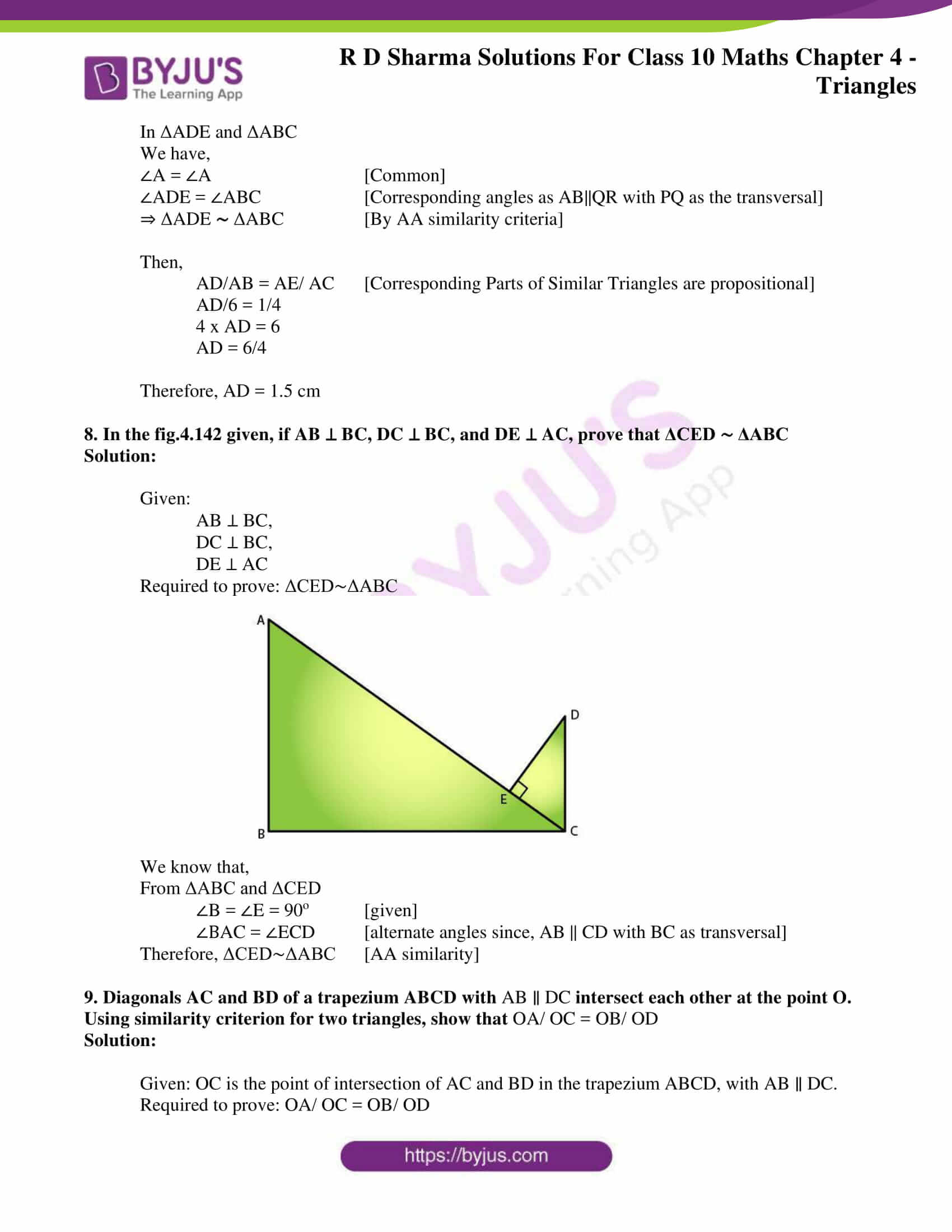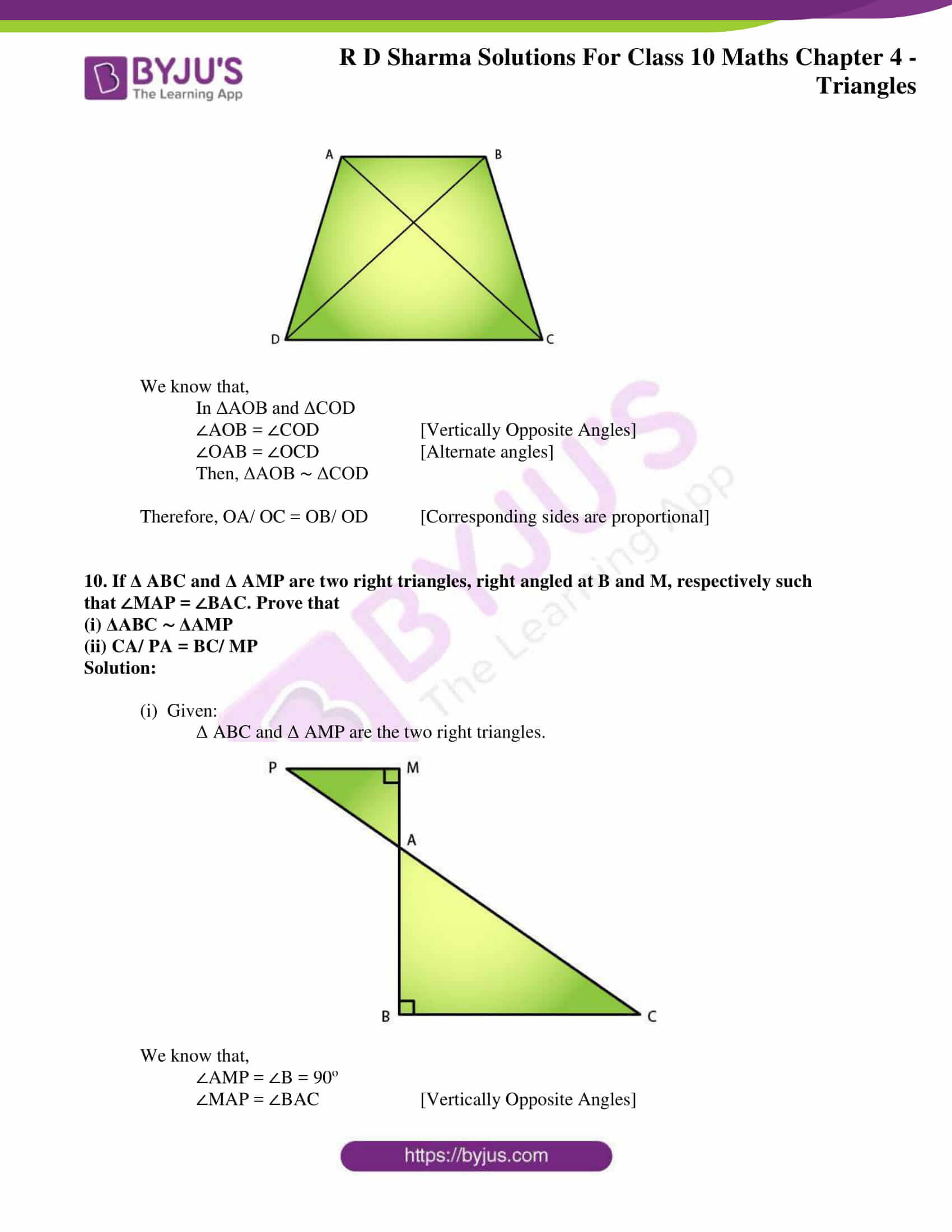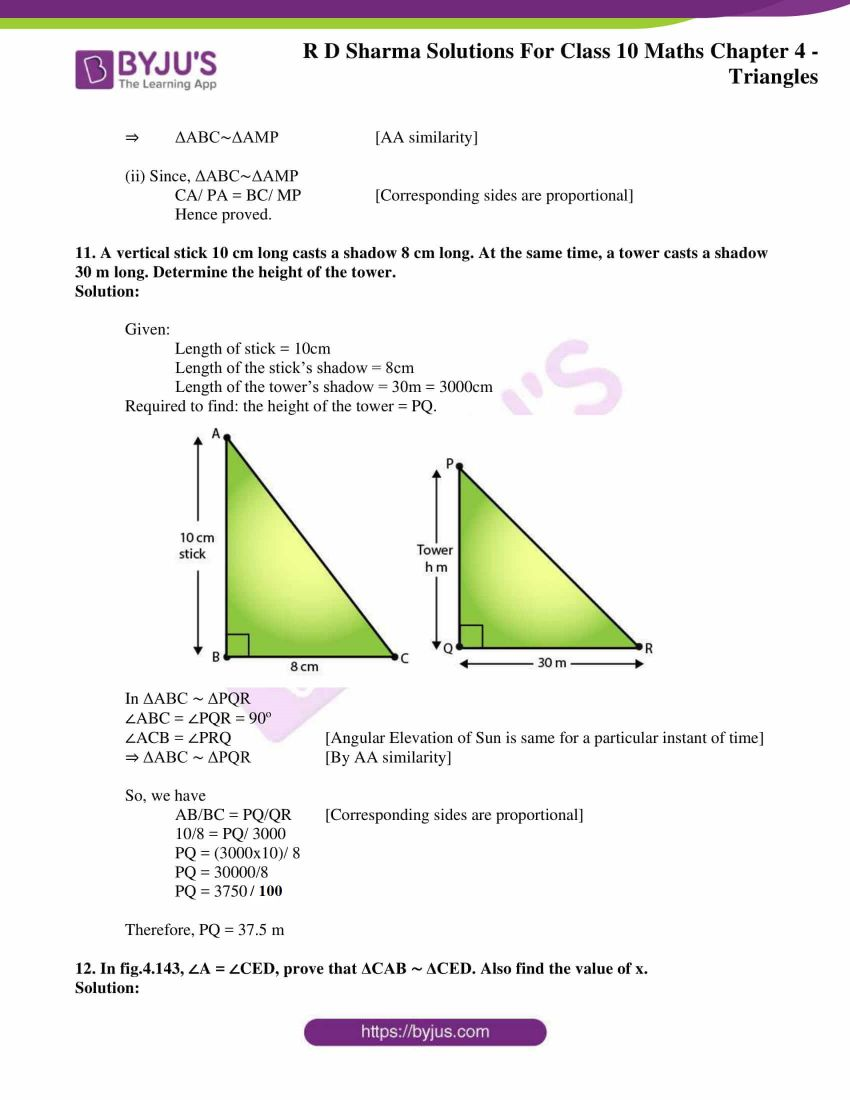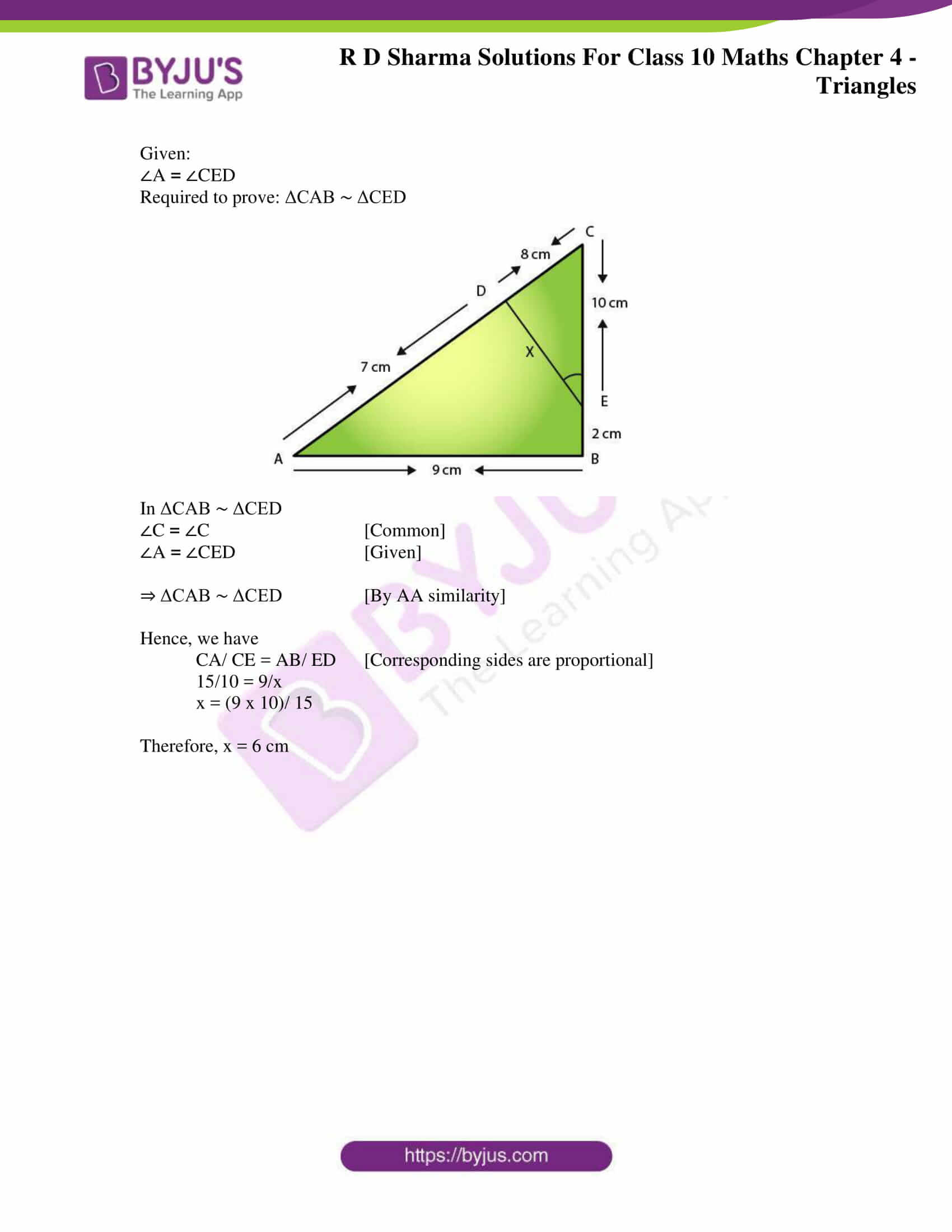### Access RD Sharma Solutions for Class 10 Maths Chapter 4 Triangles Exercise 4.5

1. In fig. 4.136, ΔACB ∼ ΔAPQ. If BC = 8 cm, PQ = 4 cm, BA = 6.5 cm and AP = 2.8 cm, find CA and AQ.

Solution:

Given,

ΔACB ∼ ΔAPQ

BC = 8 cm, PQ = 4 cm, BA = 6.5 cm and AP = 2.8 cm

Required to find: CA and AQ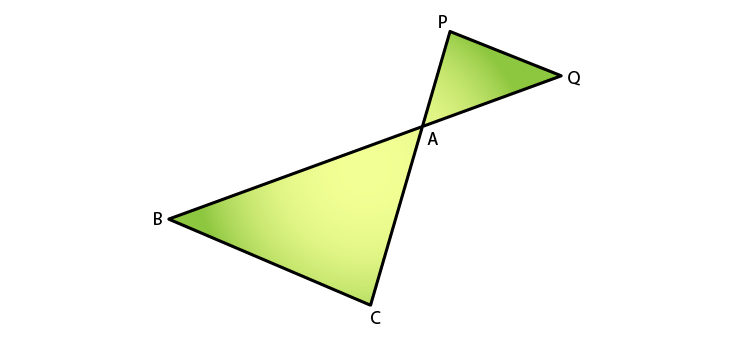We know that,

ΔACB ∼ ΔAPQ [given]

BA/ AQ = CA/ AP = BC/ PQ [Corresponding Parts of Similar Triangles]

So,

6.5/ AQ = 8/ 4

AQ = (6.5 x 4)/ 8

AQ = 3.25 cm

Similarly, as

CA/ AP = BC/ PQ

CA/ 2.8 = 8/ 4

CA = 2.8 x 2

CA = 5.6 cm

Hence, CA = 5.6 cm and AQ = 3.25 cm.

2. In fig.4.137, AB ∥ QR, find the length of PB.

Solution:

Given,

ΔPQR, AB ∥ QR and

AB = 3 cm, QR = 9 cm and PR = 6 cm

Required to find: PB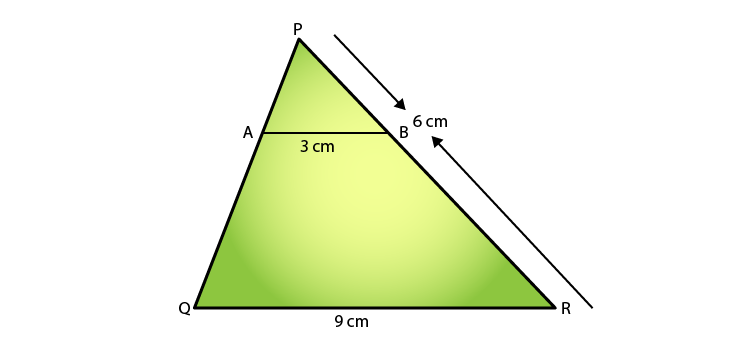In ΔPAB and ΔPQR

We have,

∠P = ∠P  [Common]

∠PAB = ∠PQR [Corresponding angles as AB||QR with PQ as the transversal]

⇒ ΔPAB ΔPQR [By AA similarity criteria]

Hence,

AB/ QR = PB/ PR [Corresponding Parts of Similar Triangles are propositional]

⇒ 3/ 9 = PB/6

PB = 6/3

Therefore, PB = 2 cm

3. In fig. 4.138 given, XY∥BC. Find the length of XY.

Solution:

Given,

XY∥BC

AX = 1 cm, XB = 3 cm and BC = 6 cm

Required to find: XY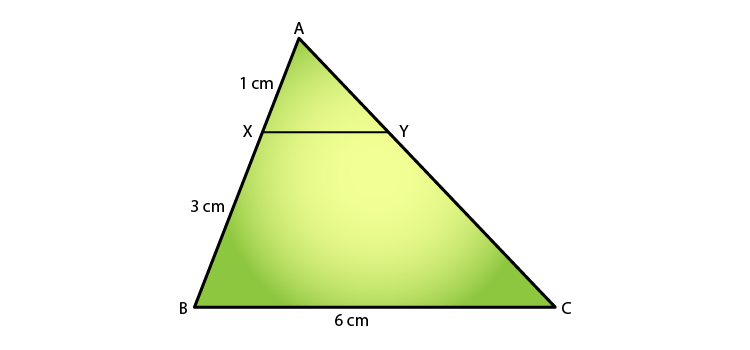In ΔAXY and ΔABC

We have,

∠A = ∠A  [Common]

∠AXY = ∠ABC [Corresponding angles as AB||QR with PQ as the transversal]

⇒ ΔAXY ΔABC [By AA similarity criteria]

Hence,

XY/ BC = AX/ AB [Corresponding Parts of Similar Triangles are propositional]

We know that,

(AB = AX + XB = 1 + 3 = 4)

XY/6 = 1/4

XY/1 = 6/4

Therefore, XY = 1.5 cm

4. In a right-angled triangle with sides a and b and hypotenuse c, the altitude drawn on the hypotenuse is x. Prove that ab = cx.

Solution:

Consider ΔABC to be a right angle triangle having sides a and b and hypotenuse c. Let BD be the altitude drawn on the hypotenuse AC.

Required to prove: ab = cx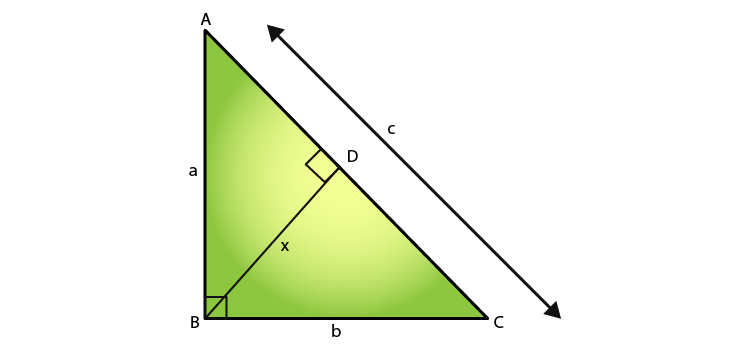We know that,

In ΔACB and ΔCDB

∠B = ∠B  [Common]

∠ACB = ∠CDB = 90o

⇒ ΔACB ΔCDB [By AA similarity criteria]

Hence,

AB/ BD = AC/ BC [Corresponding Parts of Similar Triangles are propositional]

a/ x = c/ b

⇒ xc = ab

Therefore, ab = cx

5. In fig. 4.139, ∠ABC = 90 and BD⊥AC. If BD = 8 cm, and AD = 4 cm, find CD.

Solution:

Given,

∠ABC = 90o and BD⊥AC

BD = 8 cm

Required to find: CD.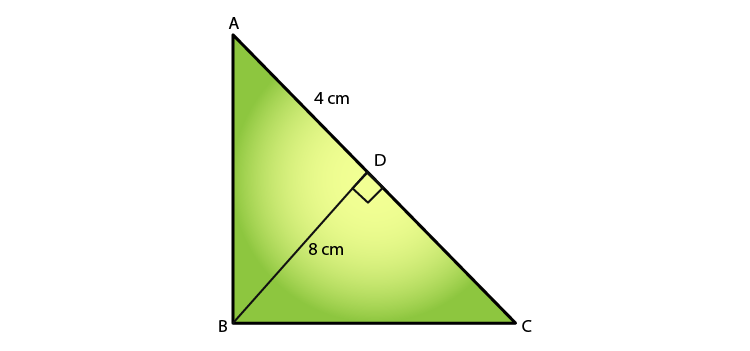We know that,

ABC is a right angled triangle and BD⊥AC.

Then, ΔDBA∼ΔDCB  [By AA similarity]

(8)2 = 4 x DC

DC = 64/4 = 16 cm

Therefore, CD = 16 cm

6. In fig.4.140, ∠ABC = 90o and BD ⊥ AC. If AC = 5.7 cm, BD = 3.8 cm and CD = 5.4 cm, Find BC.

Solution:

Given:

BD ⊥ AC

AC = 5.7 cm, BD = 3.8 cm and CD = 5.4 cm

∠ABC = 90o

Required to find: BC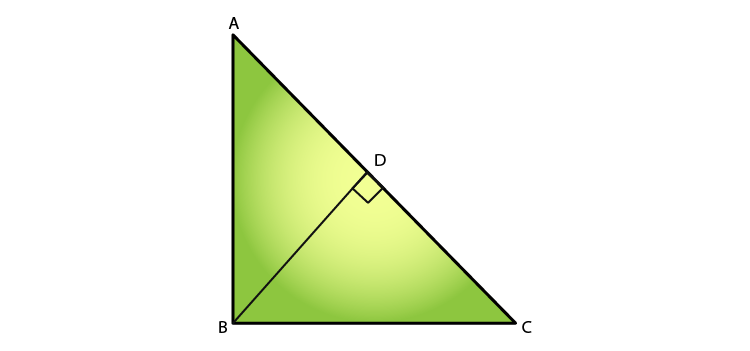We know that,

ΔABC ∼ ΔBDC [By AA similarity]

∠BCA = ∠DCA = 90o

∠AXY = ∠ABC [Common]

Thus,

AB/ BD = BC/ CD [Corresponding Parts of Similar Triangles are propositional]

5.7/ 3.8 = BC/ 5.4

BC = (5.7 x 5.4)/ 3.8 = 8.1

Therefore, BC = 8.1 cm

7. In the fig.4.141 given, DE ∥ BC such that AE = (1/4)AC. If AB = 6 cm, find AD.

Solution:

Given:

DE∥BC

AE = (1/4)AC

AB = 6 cm.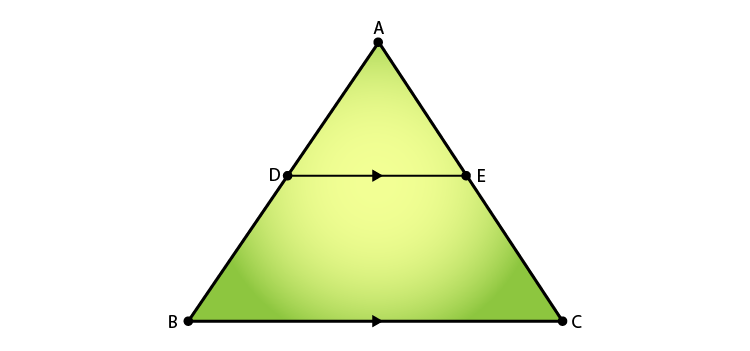We have,

∠A = ∠A  [Common]

∠ADE = ∠ABC [Corresponding angles as AB||QR with PQ as the transversal]

⇒ ΔADE ΔABC [By AA similarity criteria]

Then,

AD/AB = AE/ AC [Corresponding Parts of Similar Triangles are propositional]

8. In the fig.4.142 given, if AB ⊥ BC, DC ⊥ BC, and DE ⊥ AC, prove that ΔCED ∼ ΔABC

Solution:

Given:

AB ⊥ BC,

DC ⊥ BC,

DE ⊥ AC

Required to prove: ΔCED∼ΔABC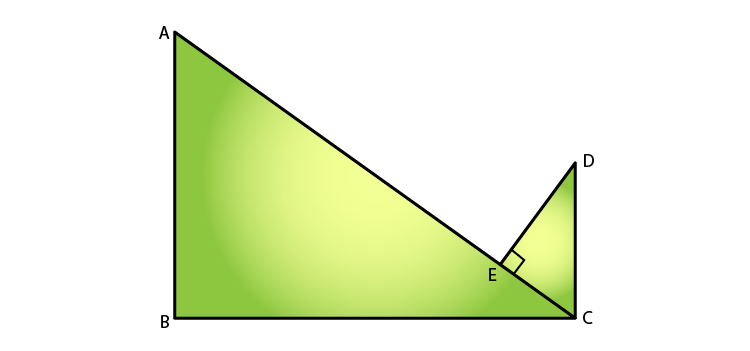We know that,

From ΔABC and ΔCED

∠B = ∠E = 90o   [given]

∠BAC = ∠ECD     [alternate angles since, AB || CD with BC as transversal]

Therefore, ΔCED∼ΔABC  [AA similarity]

9. Diagonals AC and BD of a trapezium ABCD with AB ∥ DC intersect each other at the point O. Using similarity criterion for two triangles, show that OA/ OC = OB/ OD

Solution:

Given: OC is the point of intersection of AC and BD in the trapezium ABCD, with AB ∥ DC.

Required to prove: OA/ OC = OB/ OD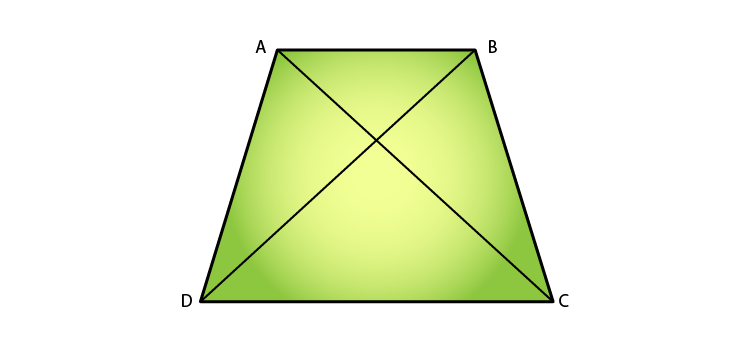We know that,

In ΔAOB and ΔCOD

∠AOB = ∠COD [Vertically Opposite Angles]

∠OAB = ∠OCD    [Alternate angles]

Then, ΔAOB ∼ ΔCOD

Therefore, OA/ OC = OB/ OD   [Corresponding sides are proportional]

10. If Δ ABC and Δ AMP are two right triangles, right angled at B and M, respectively such that ∠MAP = ∠BAC. Prove that

(i) ΔABC ∼ ΔAMP

(ii) CA/ PA = BC/ MP

Solution:

(i)  Given:

Δ ABC and Δ AMP are the two right triangles.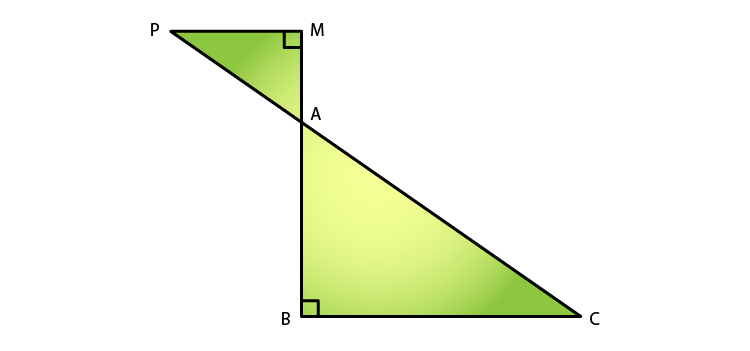We know that,

∠AMP = ∠B = 90o

∠MAP = ∠BAC [Vertically Opposite Angles]

⇒ ΔABC∼ΔAMP     [AA similarity]

(ii) Since, ΔABC∼ΔAMP

CA/ PA = BC/ MP  [Corresponding sides are proportional]

Hence proved.

11. A vertical stick 10 cm long casts a shadow 8 cm long. At the same time, a tower casts a shadow 30 m long. Determine the height of the tower.

Solution:

Given:

Length of stick = 10cm

Length of the stick’s shadow = 8cm

Length of the tower’s shadow = 30m = 3000cm

Required to find: the height of the tower = PQ.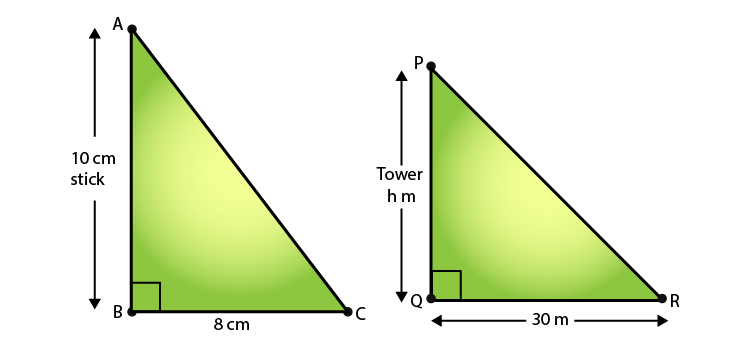In ΔABC ∼ ΔPQR

∠ABC = ∠PQR = 90o

∠ACB = ∠PRQ [Angular Elevation of Sun is same for a particular instant of time]

⇒ ΔABC ∼ ΔPQR    [By AA similarity]

So, we have

AB/BC = PQ/QR [Corresponding sides are proportional]

10/8 = PQ/ 3000

PQ = (3000×10)/ 8

PQ = 30000/8

PQ = 3750/100

Therefore, PQ = 37.5 m

12. In fig.4.143, ∠A = ∠CED, prove that ΔCAB ∼ ΔCED. Also find the value of x.

Solution:

Given:

∠A = ∠CED

Required to prove: ΔCAB ∼ ΔCED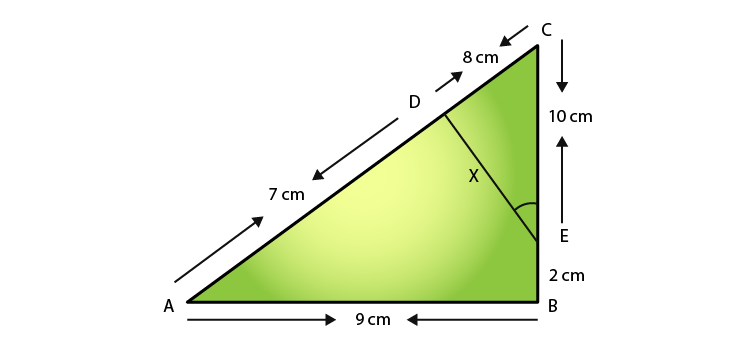In ΔCAB ∼ ΔCED

∠C = ∠C  [Common]

∠A = ∠CED [Given]

⇒ ΔCAB ∼ ΔCED [By AA similarity]

Hence, we have

CA/ CE = AB/ ED  [Corresponding sides are proportional]

15/10 = 9/x

x = (9 x 10)/ 15

Therefore, x = 6 cm